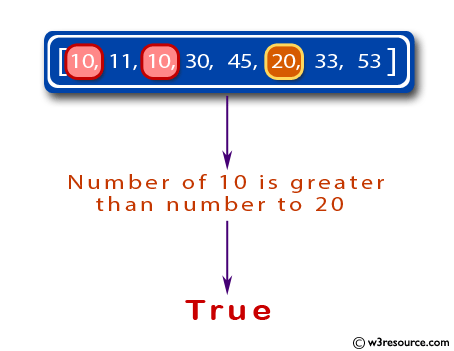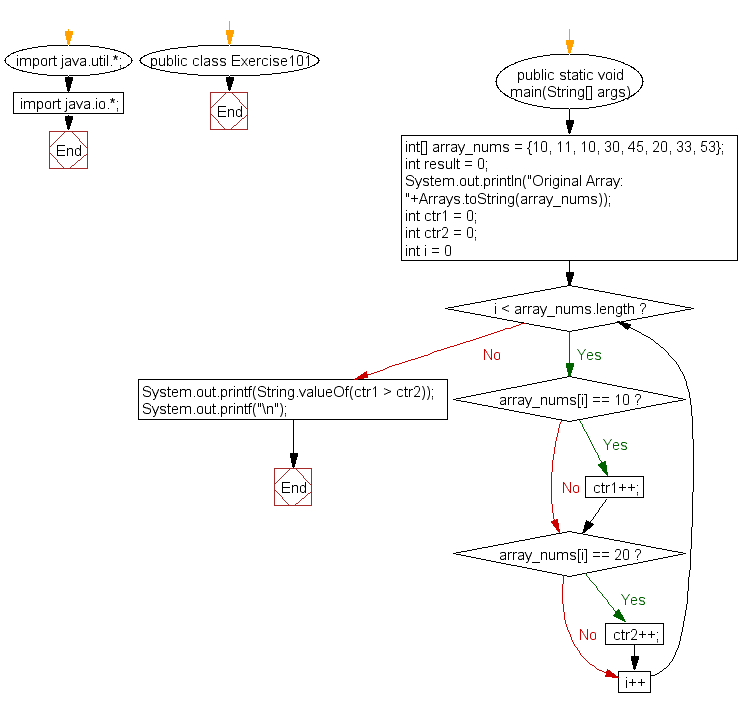﻿ Java: Check if number of 10 is greater than 20 in an array# Java Exercises: Check if the number of 10 is greater than number to 20s in a given array of integers

## Java Basic: Exercise-101 with Solution

Write a Java program to check if the number of 10 is greater than number to 20s in a given array of integers.

Pictorial Presentation:Sample Solution:

Java Code:

``````import java.util.*;
import java.io.*;
public class Exercise101 {
public static void main(String[] args)
{
int[] array_nums = {10, 11, 10, 30, 45, 20, 33, 53};
int result = 0;
System.out.println("Original Array: "+Arrays.toString(array_nums));

int ctr1 = 0;
int ctr2 = 0;

for(int i = 0; i < array_nums.length; i++) {
if(array_nums[i] == 10)
ctr1++;

if(array_nums[i] == 20)
ctr2++;
}
System.out.printf(String.valueOf(ctr1 > ctr2));
System.out.printf("\n");
}
}
```
```

Sample Output:

```Original Array: [10, 11, 10, 30, 45, 20, 33, 53]
true
```

Flowchart:Java Code Editor:

What is the difficulty level of this exercise?

Test your Programming skills with w3resource's quiz.

﻿

## Java: Tips of the Day

getEnumMap

Converts to enum to Map where key is the name and value is Enum itself.

```public static <E extends Enum<E>> Map<String, E> getEnumMap(final Class<E> enumClass) {
return Arrays.stream(enumClass.getEnumConstants())
.collect(Collectors.toMap(Enum::name, Function.identity()));
}
```

Ref: https://bit.ly/3xXcFZt